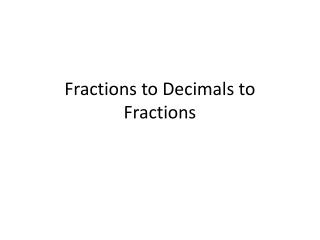DownloadDownload PresentationFractions to Decimals to Fractions

# Fractions to Decimals to Fractions

Télécharger la présentation## Fractions to Decimals to Fractions

- - - - - - - - - - - - - - - - - - - - - - - - - - - E N D - - - - - - - - - - - - - - - - - - - - - - - - - - -
##### Presentation Transcript

1. Fractions to Decimals to Fractions

2. Fractions to Decimals With a Calculator: A) Divide the top number by the bottom number (numerator by denominator) Examples:

3. B) With a Calculator * Type in Fraction * Press 2nd, PRB (F-D), = Examples:

4. Decimals to Fractions • Without a Calculator: Read it. Write exactly what you read. Examples:

5. Repeating Decimals * Write the repeating number as the numerator * Write the numerator over a series of nines * The number of nines depends on the number of repeating numbers you have in the decimal Examples:

6. 2) With a Calculator * Type in Decimal * Press 2nd, PRB (F-D), = Examples: